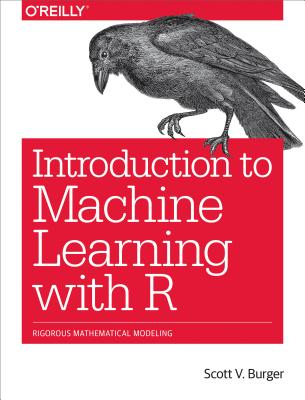Introduction to Machine Learning with R: Rigorous Mathematical AnalysisHuge savings for students

Each student receives a 50% discount off of most books in the HSG Book Store. During class, please ask the instructor about purchase details.
 List Price: \$49.99 Price: \$25.00 You Save: \$25.00
0

Machine learning is an intimidating subject until you know the fundamentals. If you understand basic coding concepts, this introductory guide will help you gain a solid foundation in machine learning principles. Using the R programming language, you'll first start to learn with regression modelling and then move into more advanced topics such as neural networks and tree-based methods.

Finally, you'll delve into the frontier of machine learning, using the caret package in R. Once you develop a familiarity with topics such as the difference between regression and classification models, you'll be able to solve an array of machine learning problems. Author Scott V. Burger provides several examples to help you build a working knowledge of machine learning.

• Explore machine learning models, algorithms, and data training
• Understand machine learning algorithms for supervised and unsupervised cases
• Examine statistical concepts for designing data for use in models
• Dive into linear regression models used in business and science
• Use single-layer and multilayer neural networks for calculating outcomes
• Look at how tree-based models work, including popular decision trees
• Get a comprehensive view of the machine learning ecosystem in R
• Explore the powerhouse of tools available in R's caret package
O'Reilly Media